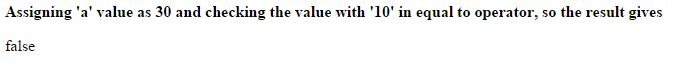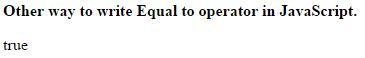# Equal (==) operator in JavaScript

##### How to check if the values of two operands are equal in JavaScript?

In the starting we learnt about Arithmetic Operators and Assignment Operators, now we shall study about the Comparison Operators in JavaScript.

### Equal (==)

Equal is an comparison operator which is used to check the value of two operands are equal or not.

If the two values are equal it returns true.

The symbolic representation of equal operator in JavaScript is `==`.

### Assigning same values

```<b>Assigning 'a' value as 30 and checking the value with '30' in equal to operator, so the result gives</b>
<p id="myId"></p>

<script>
var a = 30;
document.getElementById("myId").innerHTML = (a == 30);
</script>```

In the above code snippet we have assigned the same value i.e '30' to the variable `a` and to the equal operator. So the results gives 'true'.

OUTPUT### Assigning Different values

```<b>Assigning 'a' value as 30 and checking the value with '10' in equal to operator, so the result gives</b>
<p id="myId"></p>

<script>
var a = 30;
document.getElementById("myId").innerHTML = (a == 10); // The operator value a==10 can be written as a=="10".
</script>
```

Assigning different values

In the above code snippet we have assigned different values to the variable `a` and to the equal operator, so the result gives 'false'.

NOTE: The equal to operator value can be written as `a == 10` or `a == "10"`, both gives the same result.

OUTPUT### Basic way of using Equal to operator

```<b>Other way to write Equal to operator in JavaScript.</b>
<p id="myId"></p>

<script>
var a = 30;
var b = 30;
var c = (a == b);
document.getElementById("myId").innerHTML = c;
</script>```

Equal operator with basic program

In the above code snippet we used the simple way of writing program on equal to operator in JavaScript.

OUTPUTViews: 4058 | Post Order: 167